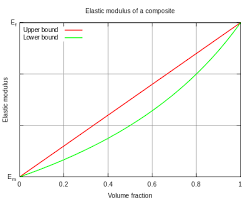## How to Calculate and Solve for Modulus of Elasticity of Composites Lower Bound | Composites

The modulus of elasticity of composites lower bound is illustrated by the image below.To compute for modulus of elasticity of composites lower bound, four essential parameters are needed and these parameters are Modulus of Elasticity of the Matrix (Em), Modulus of Elasticity of the Particle (Ep), Volume Fraction of the Matrix (Vm) and Volume Fraction of the Particle (Vp).

The formula for calculating modulus of elasticity of composites lower bound:

Ec(l) = EmEp/VmEp + VpEm

Where:

Ec(u) = Modulus of Elasticity of Composites Lower Bound
Em =Modulus of Elasticity of the Matrix
Ep = Modulus of Elasticity of the Particle
Vm = Volume Fractions of the Matrix
Vp = Volume Fractions of the Particle

Let’s solve an example;
Find the modulus of elasticity of composites lower bound when the modulus of elasticity of the matrix is 2, the modulus of elasticity of the particle is 6, the volume fractions of the matrix is 8 and the volume fractions of the particle is 4.

This implies that;

Em =Modulus of Elasticity of the Matrix = 2
Ep = Modulus of Elasticity of the Particle = 6
Vm = Volume Fractions of the Matrix = 8
Vp = Volume Fractions of the Particle = 4

Ec(l) = EmEp/VmEp + VpEm
Ec(l) = (2)(6)/(8)(6) + (4)(2)
Ec(l) = (12)/(48) + (8)
Ec(l) = (12)/(56)
Ec(l) = 0.214

Therefore, the modulus of elasticity of composites lower bound is 0.214 Pa.

## How to Calculate and Solve for Modulus of Elasticity of Composites Upper Bound | Composites

The modulus of elasticity of composites upper bound is illustrated by the image below.To compute for modulus of elasticity of composites upper bound, four essential parameters are needed and these parameters are Modulus of Elasticity of the Matrix (Em), Modulus of Elasticity of the Particle (Ep), Volume Fraction of the Matrix (Vm) and Volume Fraction of the Particle (Vp).

The formula for calculating modulus of elasticity of composites upper bound:

Ec(u) = EmVm + EpVp

Where:

Ec(u) = Modulus of Elasticity of Composites Upper Bound
Em =Modulus of Elasticity of the Matrix
Ep = Modulus of Elasticity of the Particle
Vm = Volume Fractions of the Matrix
Vp = Volume Fractions of the Particle

Let’s solve an example;
Find the modulus of elasticity of composites upper bound when the modulus of elasticity of the matrix is 4, the modulus of elasticity of the particle is 7, the volume fractions of the matrix is 2 and the volume fractions of the particle is 6.

This implies that;

Em =Modulus of Elasticity of the Matrix = 4
Ep = Modulus of Elasticity of the Particle = 7
Vm = Volume Fractions of the Matrix = 2
Vp = Volume Fractions of the Particle = 6

Ec(u) = EmVm + EpVp
Ec(u) = (4)(2) + (7)(6)
Ec(u) = (8) + (42)
Ec(u) = 50

Therefore, the modulus of elasticity of composites upper bound is 50 Pa.

Calculating the Modulus of Elasticity of the Matrix when the Modulus of Elasticity of Composites Upper Bound, the Modulus of Elasticity of the Particle, the Volume Fraction of the Matrix and the Volume Fraction of the Particle is Given.

Em = Ec(u) – EpVp / Vm

Where:

Em =Modulus of Elasticity of the Matrix
Ec(u) = Modulus of Elasticity of Composites Upper Bound
Ep = Modulus of Elasticity of the Particle
Vm = Volume Fractions of the Matrix
Vp = Volume Fractions of the Particle

Let’s solve an example;
Find the modulus of elasticity of the matrix when the modulus of elasticity of composites upper bound is 40, the modulus of elasticity of the particle is 10, the volume fractions of the matrix is 5 and the volume fractions of the particle is 2.

This implies that;

Ec(u) = Modulus of Elasticity of Composites Upper Bound = 40
Ep = Modulus of Elasticity of the Particle = 10
Vm = Volume Fractions of the Matrix = 5
Vp = Volume Fractions of the Particle = 2

Em = Ec(u) – EpVp / Vm
Em = 40 – (10)(2) / 5
Em = 40 – 20 / 5
Em = 20 / 5
Em = 4

Therefore, the modulus of elasticity of the matrix is 4.